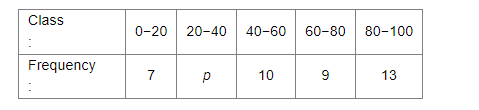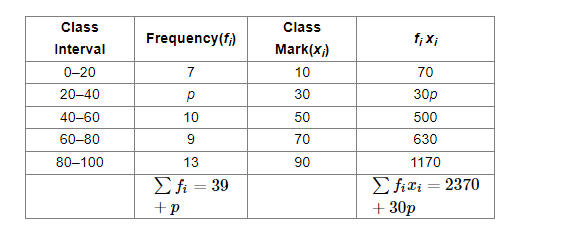# If the mean of the following distribution is 54,`
Question:

If the mean of the following distribution is 54, find the value of p:Solution:

Consider the table given below:It given that the mean of the distribution is 54.

$\therefore$ Mean $=\frac{\sum f_{i} x_{i}}{\sum f_{i}}$

$\Rightarrow \frac{2370+30 p}{39+p}=54$

$\Rightarrow 2370+30 p=2106+54 p$

$\Rightarrow 54 p-30 p=2370-2106$

$\Rightarrow 24 p=264$

$\Rightarrow p=\frac{264}{24}=11$

Hence, the value of is 11.